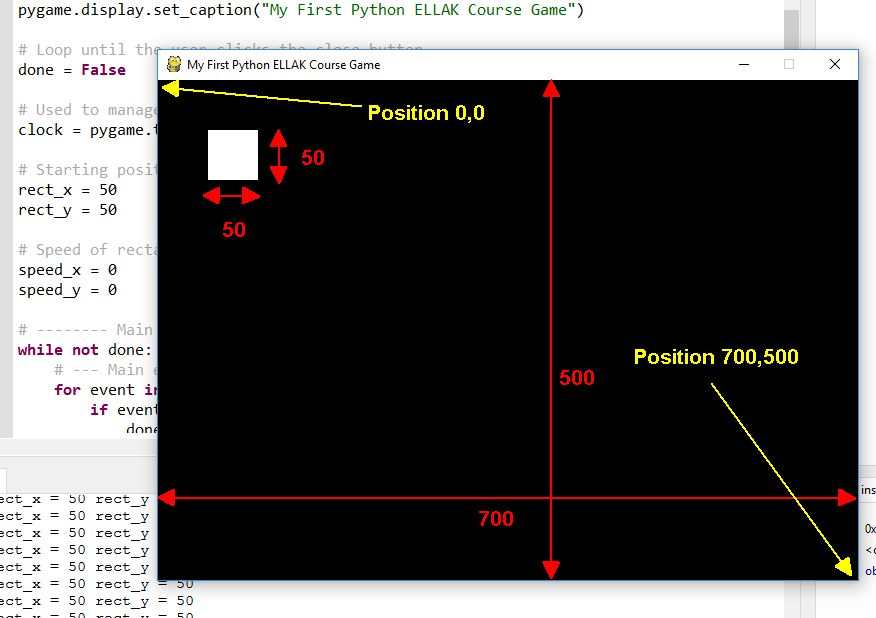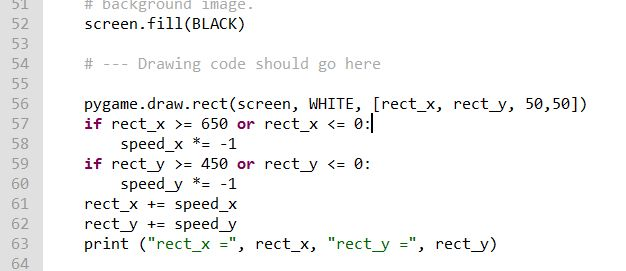### Chapter 5.5 - And stay on

5.5 - And stay on

So we managed to make the rectangle to move. Now we are going to control its movement. Download the code from here and open it to Thonny.

Change lines 33 and 34 using different integer numbers (0, 1, 2,...) and every time execute the code. Try with negative numbers (ex -5 or -1).

What happens if speed_x or speed_y is 0? What happens if they are negative?

speed = 0 means that rectangle is not moving
speed > 0 means that rectangle moves to one direction
speed < 0 means that rectangle moves to the other direction

How can we change the direction? Try this in CLI :

>>> number = 5
>>> print (number)
>>> number *= -1
>>> print(number)We will add this lines of code to the loop after drawing the rectangle (after line 56) and move the other lines downwards :

 if rect_x >= 650 or rect_x <= 0: speed_x *= -1 if rect_y >= 450 or rect_y <= 0: speed_y *= -1